# Detecting overfitting, underfitting and just right training without visualizations

I came up with a idea for an algorithm that can identify whether a model us overfitting, underfitting or is trained good enough using the loss values without visualizing them. I am not sure if this has been done before. If it has been done before, I apologize for calling it my ideaCan someone contribute some interesting losses values they’ve encountered (preferably as a pickle object) with a small description so that I can test it out?

Below, I’ve explained the algorithm with a small example and visualization.
Consider the following training loss trend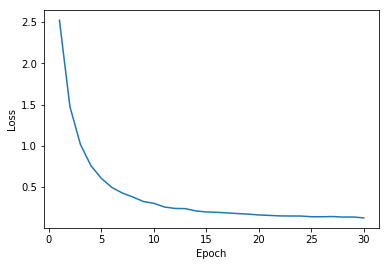The first step would be to split the losses into two halves as shown below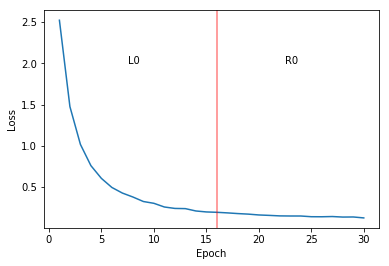Next we randomly chose a pair (x1, y1) and (x2, y2), the first one from L0 and the second one from R0 and calculate their slope. This is repeated for n times and the average of the slopes is calculated.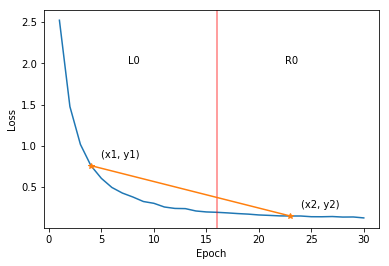In this case, the average slope calculated will most likely not be close to zero.

Now consider the following case.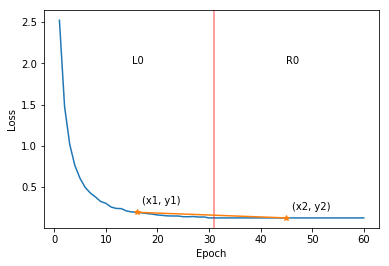Here there is is a higher chance that the mean loss will be closer to zero.
This algorithm can be extended to identify a good number of epochs. This can be done by splitting the region `L0` into two halves and calculating slopes between pontis from `L0L1` and `L0R1` as shown below.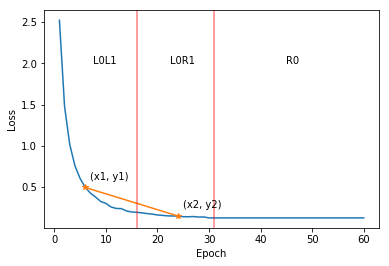The next split can be made based on the mean of the slopes calculated between the areas from the previos split. This can be done recursively and each split will give us a smaller range of epoch values.

I would love to hear other’s thoughts, suggestions on this.
All this calculation is for training loss

Edit: Now I feel using the mean alone might not be a good idea. Maybe mean and standard deviation?